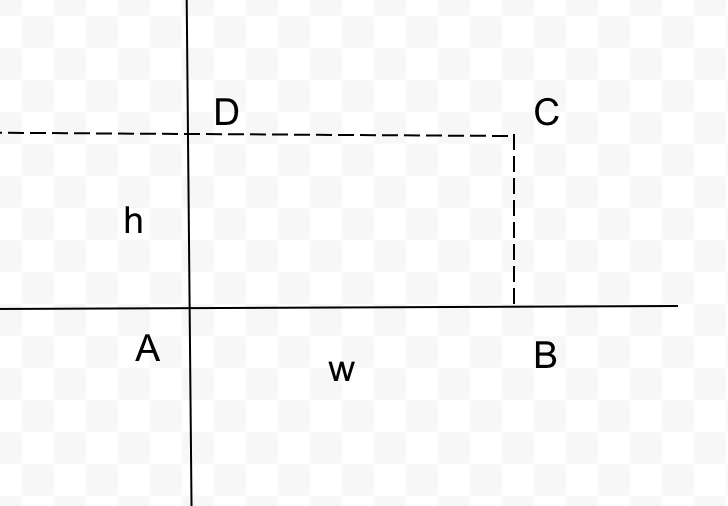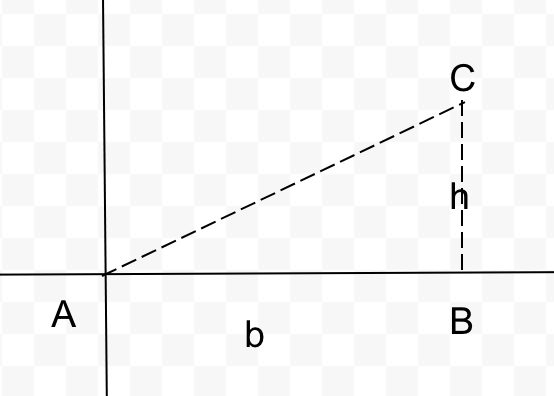Vivekanand Vellanki
2

Note sure what you are asking. There could be 2 interpretations to this question:

1. How to use integration to find area under a curve? or
2. Why does integration give the area under a curve?

It looks like you are asking about 1. The area under a curve is the definite integral of the curve. This sounds vague - lets go with a few examples.

Lets find the area of the rectangle (ABCD) in two ways. You know that the area of the rectangle is hw.Let's using integration to find the area of the following: area between the x-axis and the line y=h, from x=0 to x=w. Note that y=h represents the curve DC. The area between this curve and x-axis, bounded by x=0 to x=w is the same as the area of the rectangle ABCD.

Now, this area can be written as \int_0^wydx\ =\ \int_0^whdx.

Why is this the case? We are computing the area of the curve y=h, from x=0 to x=w. This is the definite integral given the end-points.

\int_0^whdx=hw\ -\ h0\ =\ hw, the area of the rectangle as expected.

Lets look at a triangle now.The area of the triangle ABC = \frac{1}{2}bh. Lets see if integration gives us the same.

We first need to find the equation of the curve (AC) and then compute the definite integral of the curve from 0 to b.

The equation of AC is y=\frac{h}{b}x. The slope is h/b and the y-intercept is 0.

The area of ABC is \int_0^bydx=\int_0^b\frac{h}{b}xdx=\frac{h}{b}\int_0^bxdx=\frac{h}{b}\times\frac{1}{2}\left(b^2-0^2\right)=\frac{1}{2}\times\frac{h}{b}\times b^2=\frac{1}{2}bh

Essentially, the definite integral of the curve from a to b represents the area under the curve and x-axis from x=a to x=b.

This applies to any curve - even 3-d dimensional surfaces. Try with a trapezium and see if that works.